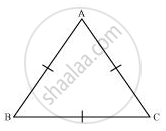Share

# Show that the Angles of an Equilateral Triangle Are 60º Each. - CBSE Class 9 - Mathematics

ConceptProperties of a Triangle

#### Question

Show that the angles of an equilateral triangle are 60º each.

#### SolutionLet us consider that ABC is an equilateral triangle.

Therefore, AB = BC = AC

AB = AC

⇒ ∠C = ∠B (Angles opposite to equal sides of a triangle are equal)

Also,

AC = BC

⇒ ∠B = ∠A (Angles opposite to equal sides of a triangle are equal)

Therefore, we obtain

∠A = ∠B = ∠C

In ΔABC,

∠A + ∠B + ∠C = 180°

⇒ ∠A + ∠A + ∠A = 180°

⇒ 3∠A = 180°

⇒ ∠A = 60°

⇒ ∠A = ∠B = ∠C = 60°

Hence, in an equilateral triangle, all interior angles are of measure 60º.

Is there an error in this question or solution?

#### APPEARS IN

NCERT Solution for Mathematics Class 9 (2018 to Current)
Chapter 7: Triangles
Ex. 7.20 | Q: 8 | Page no. 124

#### Video TutorialsVIEW ALL 

Solution Show that the Angles of an Equilateral Triangle Are 60º Each. Concept: Properties of a Triangle.
S